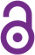Effects of porosity on seismic velocities, elastic moduli and Poisson's ratios of solid materials and rocks

Chengbo Yu, Shaocheng Ji and Qi Li

Article (2016)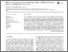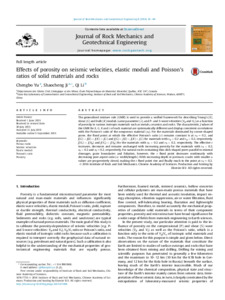Preview
Published Version
 Cite this document: Yu, C., Ji, S. & Li, Q. (2016). Effects of porosity on seismic velocities, elastic moduli and Poisson's ratios of solid materials and rocks. Journal of Rock Mechanics and Geotechnical Engineering, 8(1), p. 35-49. doi:10.1016/j.jrmge.2015.07.004

Abstract

The generalized mixture rule (GMR) is used to provide a unified framework for describing Young's (E), shear (G) and bulk (K) moduli, Lame parameter (λ), and P- and S-wave velocities (Vp and Vs) as a function of porosity in various isotropic materials such as metals, ceramics and rocks. The characteristic J values of the GMR for E, G, K and λ of each material are systematically different and display consistent correlations with the Poisson's ratio of the nonporous material (ν0). For the materials dominated by corner-shaped pores, the fixed point at which the effective Poisson's ratio (ν) remains constant is at ν0 = 0.2, and J(G) > J(E) > J(K) > J(λ) and J(G) < J(E) < J(K) < J(λ) for materials with ν0 > 0.2 and ν0 < 0.2, respectively. J(Vs) > J(Vp) and J(Vs) < J(Vp) for the materials with ν0 > 0.2 and ν0 < 0.2, respectively. The effective ν increases, decreases and remains unchanged with increasing porosity for the materials with ν0 < 0.2, ν0 > 0.2 and ν0 = 0.2, respectively. For natural rocks containing thin-disk-shaped pores parallel to mineral cleavages, grain boundaries and foliation, however, the ν fixed point decreases nonlinearly with decreasing pore aspect ratio (α: width/length). With increasing depth or pressure, cracks with smaller α values are progressively closed, making the ν fixed point rise and finally reach to the point at ν0 = 0.2.

Uncontrolled Keywords

Porous rocks ; Seismic velocities ; Elastic moduli ; Poisson's ratio ; Porosity

 Subjects: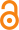Open Access document in PolyPublie 1400 Génie minier et minéral > 1400 Génie minier et minéral Département des génies civil, géologique et des mines Non applicable Natural Sciences and Engineering Council of Canada 17 Jan 2019 14:23 18 Jan 2019 01:20 https://publications.polymtl.ca/3615/
 Journal Title: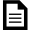Document issued by the official publisher Journal of Rock Mechanics and Geotechnical Engineering (vol. 8, no. 1) Elsevier https://doi.org/10.1016/j.jrmge.2015.07.004